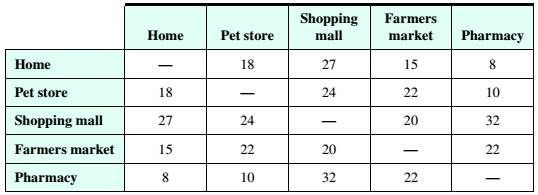# Route Planning Brian needs to visit the pet store, the shopping mall, the local farmers market, and the pharmacy. His estimated driving times (in minutes) between the locations are given in the table below. Use the greedy algorithm and the edge-picking algorithm to find two possible routes, starting and ending at home, that will help Brian minimize his total travel time.### Mathematical Excursions (MindTap C...

4th Edition
Richard N. Aufmann + 3 others
Publisher: Cengage Learning
ISBN: 9781305965584

#### Solutions

Chapter
Section### Mathematical Excursions (MindTap C...

4th Edition
Richard N. Aufmann + 3 others
Publisher: Cengage Learning
ISBN: 9781305965584
Chapter 5.2, Problem 27ES
Textbook Problem
132 views

## Route Planning Brian needs to visit the pet store, the shopping mall, the local farmers market, and the pharmacy. His estimated driving times (in minutes) between the locations are given in the table below. Use the greedy algorithm and the edge-picking algorithm to find two possible routes, starting and ending at home, that will help Brian minimize his total travel time.To determine

To find two possible routes, starting and ending at home, that will help Brain minimize his total travel time, using the greedy algorithm and the edge-picking algorithm.

### Explanation of Solution

Given information:

Given, Brian needs to visit the pet store, the shopping mall, the local farmers market, and the pharmacy.

His estimated driving times (in minutes) between the locations are given in the table below:

His starting and ending points are home and needs to have a minimum total travel time.

Calculation:

Given, there are five locations to visit which starts and ends at Home.

Hence five vertices corresponding to the five locations are marked with respective names.

And then making the complete graph by marking every possible edge with every edge marked with the weight represented in the table between the locations.

The driving time between Home and Pet store is 18. Hence the edge drawn between them has a weight of 18.

Similarly marking all the edges with respective weights, the graph is given as:

Greedy algorithm:

To use the greedy algorithm, the graph must be Hamiltonian.

(Since, Brian needs to visit each location only once)

A path in a Hamiltonian graph is said to be a Hamiltonian Circuit if it begins and ends at the same vertex and passes through each vertex of a graph exactly once.

The greedy algorithm states to travel along a connected edge that has the smallest weight that is not yet visited. This process continues till all the vertices are visited and returned to the starting vertex.

Here, the vertex representing Home is selected.

Vertex representing Home is of degree 4 and the weights of the connected edges are 18, 27, 15, 8

Hence, as per the Greedy Algorithm the edge with weight 8 is selected i.e. path reaches vertex representing Pharmacy.

Vertex representing Pharmacy is of degree 4 and the weights of the connected edges are 8, 10, 32, 22

The least of the weights is 8 but it leads to vertex representing Home which is already visited. Hence, as per the Greedy Algorithm the edge with weight 10 is selected i.e. path reaches vertex representing Pet Store.

Vertex representing Pet Store is of degree 4 and the weights of the connected edges are 18, 24, 22, 10

The least of the weights is 10 but it leads to vertex representing Pharmacy which is already visited. The next least of the weights is 18 but it leads to vertex representing Home which is already visited.

Hence, as per the Greedy Algorithm the edge with weight 22 is selected i.e. path reaches vertex representing Farmers market.

Vertex representing Farmers market is of degree 4 and the weights of the connected edges are 15, 22, 20, 22

The least of the weights is 15 but it leads to vertex representing Home which is already visited.

Hence, as per the Greedy Algorithm the edge with weight 20 is selected i.e. path reaches vertex representing Shopping mall.

Vertex representing Shopping mall is of degree 4 and the weights of the connected edges are 27, 24, 20, 32

The least of the weights is 20 but it leads to vertex representing Farmers market which is already visited...

### Still sussing out bartleby?

Check out a sample textbook solution.

See a sample solution

#### The Solution to Your Study Problems

Bartleby provides explanations to thousands of textbook problems written by our experts, many with advanced degrees!

Get Started

Find more solutions based on key concepts
Fill in the blanks. 8. a. The statement limxf(x) = L means that f(x) can be made arbitrarily close to _________...

Applied Calculus for the Managerial, Life, and Social Sciences: A Brief Approach

A population with a mean of = 8 has SX = 40. How many scores are in the population?

Statistics for The Behavioral Sciences (MindTap Course List)

Solve each equation and check: 14b=57

Elementary Technical Mathematics

Sketching a Polar Graph In Exercises 81-92, sketch a graph of the polar equation. r2=4sin

Calculus: Early Transcendental Functions (MindTap Course List)

True or False: If f′(x) = g′(x), then f(x) = g(x).

Study Guide for Stewart's Single Variable Calculus: Early Transcendentals, 8th

In Problems 710 the independent variable x is missing in the given differential equation. Proceed as in Example...

A First Course in Differential Equations with Modeling Applications (MindTap Course List)

1. Consider the following time series data. Week 1 2 3 4 5 6 Value 18 13 16 11 17 14 Using the naive method (m...

Modern Business Statistics with Microsoft Office Excel (with XLSTAT Education Edition Printed Access Card) (MindTap Course List)

Sketching Graphs In Exercises S-26 through S-35, sketch a graph with the given properties. We note that for eac...

Functions and Change: A Modeling Approach to College Algebra (MindTap Course List)